2.10 Division of Polynomials

We have learnt the following statement to be true for any number

Dividend = (divisor*quotient) + remainder.

The above relationship holds good for polynomials also.

2.10.1 Division of Monomial by monomial

2.10.1 Problem 1: Divide 12m3 n5    by 4 m2 n

Solution:

Step 1: 12m3 n5 / 4 m2 n =  (12/4)* (m3 n5 /m2 n)

Step 2:

12/4 =   3,

Step 3:

m3 n5/ m2 n = m3-2 n5-1 = m n412m3 n5 /4 m2 n = 3 m n4

Verification:

Divisor*quotient + reminder = 4 m2 n*3 m n4 +0 =12 m2+1 n1+4 =12m3 n5   which is dividend

2.10.1 Problem 2 : Divide 57x2y2z2  by 19xyz

Step 1 :

57x2y2z2 /19xyz  = (57/19) * (x2y2z2)/xyz

Step 2:

57/19 =3

Step 3:

x2y2z2/xyz = x2-1y2-1z2-1  = xyz

Thus 57x2y2z2 /19xyz  = (57/19) * (x2y2z2)/xyz  =3xyz

Verification:

(Divisor*Quotient) + Remainder = (3xyz * 19xyz) +0 = (3*19)*xyz*xyz +0=   57x1+1y1+1z1+1+0=57x2y2z2   which is dividend!

We observe 3 is quotient of 57/19 which is nothing but quotient of coefficients of monomials (57 and 19)

Similarly xyz is quotient of x2y2z2 /xyz which is nothing but quotient of the variables (x2y2z2 and xyz)

Steps to divide a monomial by monomial:

The quotient has two parts – coefficient and variable. How do we get these?

1. The coefficient of quotient of two monomials is equal to the quotient of their coefficients

2. The variable part in the quotient of two monomials is nothing but the quotient of the variables in the monomials

2.10.2 Division of a Polynomial by a Monomial

2.10.2 Problem 1: Divide 4023m2n2-6032m2n -8042m3 n4 by (-2012m2)

Solution:

We know that

4023= (2x201)3= (2)3x(201)3, 6032  = (3x201)2 = (3)2x(201)2, 8042  = (4x201)2 = (4)2x(201)2[4023m2n2-6032m2n -8042m3 n4]/(-2012m2)

=[(2)3*(201)3 m2n2-(3)2*(201)2 m2n -(4)2*(201)2m3 n4]/(-2012m2)

= -[ (2)3*(201) n2-(3)2* n -(4)2*m1 n4] = - (8*201* n2-9n -16mn4)

Verification:

Divisor*quotient + reminder

= (-2012m2)*[-(8*201* n2+9n +16mn4)]+0=

= +(2012m2)*(8*201* n2 -2012m2*9n -2012m2*16mn4) +0

= 8*2013m2 n2  -9*2012m2+2n-16*2012m2+1n4)

= 23*  2013m2 n2  - 32 *2012m4n-42*2012 m3 n4

= (2*201)3m2n2-(3*201)2 m2n –(4*201)2 m3 n4

= 4023 m2n2 - 6032 m2n - 8042 m3 n4

= dividend

2.10.2 Problem 2 :  Divide 2a4 b3+ 8a2 b2   by 2ab

Solution:

(2a4 b3+ 8a2 b2)/2ab = (2a4 b3/2ab) + (8a2 b2 / 2ab) = a3 b2 +4a b

Verification:

Divisor*quotient + reminder = 2ab*(a3 b2 +4a b) +0= 2a4 b3+ 8a2 b2   which is dividend

Steps to divide a polynomial by the monomial:

1. Divide each term of the polynomial by the monomial.

2. The partial quotients when expressed collectively become the quotient of polynomial.

2.10.3 Division of a Polynomial by a Binomial/Trinomial (Long division method)

2.10.3 Problem 1: To begin with let us learn the steps of division by dividing 7+x3-6x (a trinomial) by x+1(a binomial)

Solution:

Note degree of dividend(x3 -6x+7)   is 2 and degree of divisor(x+1)  is 1

 Step Procedure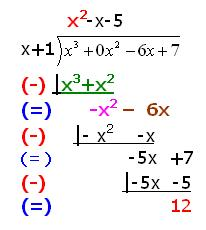1 Arrange  the terms of dividend and divisor in descending order of their degrees(Already in descending order) 2 If any term of a degree is missing in dividend or divisor, add that degree with coefficient as 0. Write dividend  x3  -6x+7  as x3  +0x2-6x+7 3 Divide the first term of the dividend by the first term of the divisor,( x3/x = x2)  Hence, x2  is the first term of the quotient, write this term on the top of the dividend. 4 Multiply divisor(which is x+1) by the first term of the quotient(which is x2) and write the product(=x3+ x2) below the dividend 5 Subtract result of step 4 from the given dividend. The result is ( x3 +0x2 ) – (x3+ x2) =  - x2 6 Take term of next degree (=-6x) from the given dividend and write it next to the result got in step 5. The result is -x2 – 6x. Consider this as new dividend 7 Repeat steps 3 to 6, by taking terms from given dividend  in step corresponding to step 6 8 Repeat above procedure till the degree of reminder is less than degree of divisor

Verification:

Divisor *Quotient +Reminder = (x+1)* (x2-x-5)+12  = x*(x2-x-5) +1*(x2-x-5)+12 = (x3-x2-5x)+ (x2-x-5)+12 = x3-x2+ x2-5x-x -5+12 = x3-0x2-6x +7 = x3-6x +7

which is nothing but dividend

2.10.3 Problem 2: x5 -9x2 +12x-14 divided by x -3

Solution:

Though, the dividend is in descending order of power of x, we need to have missing terms of powers of x (x4 ,x3). This is done by having their co-efficients as zero.

The dividend is re written as  x5 +0x4 +0x3-9x2 +12x-14. The divisor is already in descending order of power of x.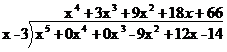- | x5 -3x4

-    |3x4 +0x3

- |3x4 -9x3

-  |9x3 -9x2

- |9x3 -27x2

-  |18x2+12x

-  |18x2 -54x

-|66x-14

-|66x-198

184

Verification:

We can verify the solution by doing proper multiplication of terms.

Since terms are big, let us verify by an alternative method of substitution.

Let us find the results when x=2

Then

Dividend =x5 -9x2 +12x-14 = 25 -9*22 +12*2-14 = 32-36+24-14 = 6

Divisor = x-3 =2-3 = -1

Quotient =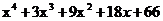= 24 +3*23 +9*22+18*2+66 = 16+24+36+66=178

Quotient*Divisor + Reminder = 178*-1+184 = -178+184= 6 which is dividend

2.10.3 Problem 3:  Divide 6p3 -19p2 -8p by p2 -4p+2

Solution:

6p+5

p2 -4p+2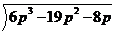( -)     |6p3 -24p2 +12p                --à ---- (1)      {= 6p*(p2 -4p+2)}

(=)             |+5 p2 -20p                --à -----(2)     {subtract (1) from  given dividend}

( -)             | 5p2 - 20p+10           --à -----(3)      {= 5*(p2 -4p+2)}

(=)                              -10           --à                 {subtract (3) from (2)}

Verification:

Quotient *Divisor = (6p+5)* (p2 -4p+2) = 6p* p2  +6p*-4p+6p*2+5* p2+5*-4p+5*2 = 6p3 -24p2+12p+5p2-20p+10

=6p3 -19p2-8p+10

Quotient *Divisor + Reminder = (6p3 -19p2-8p+10)-10 = 6p3 -19p2-8p which is dividend

2.10.3 Problem 4: Divide   a5 +b5 by a+b

Solution:

a+b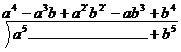(-)  |a5+ a4b

(=)   - a4b+0

(-) |a4b-a3b2

(=)       a3b2+0

(-) | a3b2+ a2b3

(=)           - a2b3+0

(-) |-a2b3-ab4

(=)            ab4 + b5

(-)    |ab4 + b5

(=)         0

Exercise : Verify that Divisor*Quotient+ Reminder = dividend

2.10 Summary of learning

 No Points studied 1 Division of algebraic expressions

Additional points:

Synthetic method (Horner’s method) of division when the divisor is of the form x-a.

We shall describe this method by taking the problem which was worked out earlier (2.10.3 Problem 2).

Divide x5 -9x2 +12x-14 by x -3

Solution:

Write the dividend in its standard form as: 1x5 + 0x4 + 0x3 - 9x2 + 12x - 14.

Here the constant term in the divisor is -3

First, write the –negative of constant term in the divisor (3 in this case) in the first column of the first row. In the next columns of the first row write the co-efficients of the dividend (1, 0, 0, -9, 12, -14)

Write the co-efficient of the first term of the divisor (in this case 1) in the corresponding column of the third row down below (in 2nd column).

starting from this column in the third row, write the product of divisor (in this case 3) and the number in this column (in this case 1) in the next column of 2nd row (in this case 3*1=3 in the 3rd column). Add these numbers in the 1st and 2nd row (in this case 0+3=3) into the corresponding column in the third row. Repeat this process till the result in the last column in the third row is got. The value in the last column of the third row gives the reminder.

 Divisor Dividend portion 3 1 0 0 -9 12 -14 First Row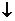3(=3*1) 9(= 3*3) 27(= 3*9) 54(= 3*18) 198(= 3*66) Second row 1 3=(0+3) 9(= 0+9) 18(=-9+27) 66(=12+54) 184(=-14+198) Third row

You will observe that the reminder is 184, which is same as what we got while solving problem (2.10.3 Problem 2)

Roots of an equation:

Let us take the polynomial 4023m2n2 - 6032m2n - 8042m3 n4.

Since this polynomial contains m and n as variables we can denote the same by f(m,n).

f(m,n) is pronounced as ‘function of m and n’.

f(m,n) = 4023m2n2 - 6032m2n - 8042m3 n4

A polynomial f(x) in one variable x is an algebraic expression of the form

f(x) = anxn+ an-1xn-1+ an-2xn-2+ ………. a2x2+ a1x+ a0 = 0

where a0,a1,a2,……… an-1,an are constants and an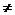0

a0,a1,a2,……… an-1 and an are called ‘co-efficients’ of  x0,x1,x2……. xn-1 and xn respectively. n is called the ‘degree’ of the polynomial.

Each of anxn, an-1xn-1,………. a2x2, a1x1, a0 are called the ‘terms’ of the polynomial.

Let f(x) = x5 - 9x2 + 12x - 14

If we substitute x = 0 we get f(0) =  0 -9*0 +12*0 -14 = -14

If we substitute x = 1 we get f(1) =   1-9+12-14= -10

Similarly, if we substitute x = -1 we get f(-1) =  -36

f(a) = a5 - 9a2 + 12a - 14

If for any value of a (x=a), f(x) = 0, then we say that ‘a’ is a ‘root’ of the equation f(x)=0.

2.10.3 Problem 5: Check if 0, 1, 2 are roots of the equation x2-2x=0

Solution:

Let f(x) = x2-2x

We note that f(0) = 02-2*0 = 0,

f(1) = 12-2 = -1

f(2) = 22-2*2 = 0

Thus 0 and 2 are the roots of the given polynomial but 1 is not.

2.10.3 Problem 6: If f(x) = x2+5x+p and q(x) = x2+3x+q have a common factor then

(i) Find the common factor

(ii) Show that (p-q)2= 2(3p-5q)

Solution:

Since degree of f(x) is 2 and it has a common factor, the degree of the factor has to be one.

Let it be x-kf(k) = k2+5k+p = 0

Since x-k is also a factor of q(x)q(k) = k2+3k+q = 0k2+5k+p = k2+3k+q: On simplificationk = (1/2)(q-p)

Hence the common factor = x-k = x - (1/2)(q-p)

= x + (1/2)(p-q)

By substituting the value of k in f(x) we get

((q-p)/2)2+5(q-p)/2+p = 0

i.e. (p-q)2/4+5(q-p)/2+p = 0

i.e. (p-q)2+10(q-p)+4p = 0

i.e. (p-q)2 = 10p-10q-4p

= 6p-10q

= 2(3p-5q)